Electron. J. Differential Equations, Vol. 2019 (2019), No. 87, pp. 1-20.

### Existence of infinitely many solutions of p-Laplacian equations in R^N+ Junfang Zhao, Xiangqing Liu, Jiaquan Liu

Abstract: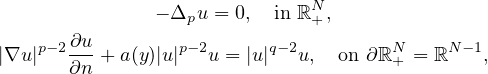where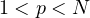,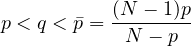,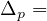div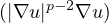the p-Laplacian operator, and the positive, finite function a(y) satisfies suitable decay assumptions at infinity. By using the truncation method, we prove the existence of infinitely many solutions.Junfang Zhao School of Science China University of Geosciences Beijing 100083, China email: zhao_junfang@163.com Xiangqing Liu Department of Mathematics Yunnan Normal University Kunming 650500, China email: lxq8u8@163.com Jiaquan Liu LMAM, School of Mathematics Peking University Beijing 100871, China email: jiaquan@math.pku.edu.cn